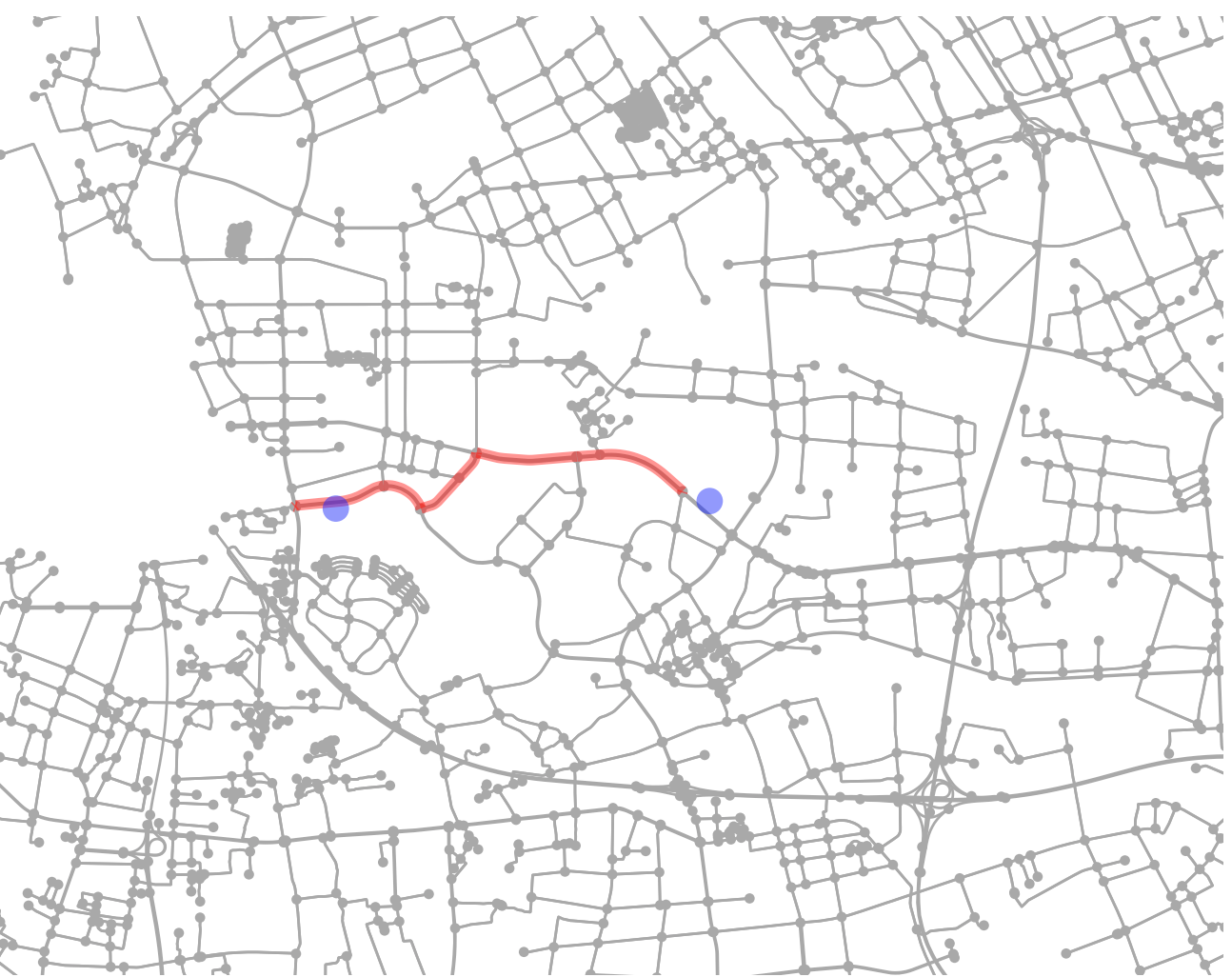4000*4000/30000=533，也就是我要533天才能把我的矩阵算出来。这显然行不通啊，500天项目早就黄了。然后去网上搜了半天，怎么绕开调用限制，基本没有很好的解决方法，仅仅在官网上找到这个：

2万块钱我做完这个项目都赚不到。。没办法，于是就想到了OpenStreetMap。OpenStreetMap基本接触过地图处理的人都有听过，一个完全开源免费的地图库，做开发处理都非常强大。现将整个过程整理如下，希望大家能少走弯路。

``````conda create --override-channels -c conda-forge -n OSMNX python=3 osmnx
source activate OSMNX``````

``````import osmnx as ox
import networkx as nx
import os``````

``````G = ox.graph_from_place('Shanghai, China', which_result=2, network_type='drive')
fig, ax = ox.plot_graph(ox.project_graph(G))
ox.save_graphml(G, filename='STREETGRAPH_FILENAME')``````

``````origin_point = (31.2827320285,121.2106132507)
destination_point = (31.2819500000,121.1651300000)
origin_node = ox.get_nearest_node(G, origin_point)
destination_node = ox.get_nearest_node(G, destination_point)
route = nx.shortest_path(G, origin_node, destination_node, weight='length')
str(route)
distance = nx.shortest_path_length(G, origin_node, destination_node, weight='length')
fig, ax = ox.plot_graph_route(G, route, origin_point=origin_point, destination_point=destination_point)``````